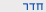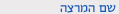שנה"ל תש"ףתכונות פיסיקליות של גבישים
Physical Properties of Crystals
0581-4232
הנדסה | תואר שני - מדע והנדסת חומרים
קבוצה 01סמ'  א'1200-1500130 ולפסון הנדסהשיעור ד"ר גורפמן סמיון
דרישות קדם   בחינה   רשימת התפוצה
הקורס מועבר באנגלית
ש"ס:  3.0

סילבוס מקוצר

Elective course: Physical properties of crystals

First semester, 2019 – 2020

Lecturer:

Dr Semën Gorfman

(129, Wolfson Building for Mechanical Engineering, gorfman@tauex.tau.ac.il)

Language:

English

The goal of this course:

To become familiar with the physical properties of crystalline materials, their description and applications.

Prerequisites:

All the basic courses in mathematics and physics.

Introduction to crystallography and structure analysis.

Format of the course:

3 hours per week of lecture (including some examples exercises, solved in the classroom). You will get homework assignments to be submitted individually.

The final grade will be calculated from the exam (80 %) and the solved homework (20%). However, many additional extra-tasks will be available throughout the course and there will be opportunity to collect sufficient number of points during the semester.

Tentative topics:

Part I. Mathematical Introduction

Introduction: Definition of materials properties. Physical properties of crystals.

Operations with tensors: The use of tensors for the description of materials properties. Transformation of coordinate systems and tensor components.

Symmetry: Definition of symmetry, symmetry operations, mathematical representation of symmetry operations. The role of symmetry of the structures for materials properties. Neumann principle.

Symmetry and physical properties of crystals: Symmetry of crystals. Bravais types of lattices, crystal systems and point symmetry groups.

Part II. Physical properties of materials

Electrocaloric and dielectric properties of crystals:  Heat capacity, pyroelectric effect, electrocaloric effect and dielectric susceptibility.  Polar and non-polar crystals. Applications.

Strain and stress tensors: Description of materials deformation and external forces by second rank tensors. Tensile and shear strains / stresses.

Elastocaloric properties of crystals: Thermal expansion and piezocaloric effect. Thermodynamics of elastocaloric properties and their relation to crystal symmetry. Negative thermal expansion coefficients. Preparation of cuts for zero thermal expansion.

Piezoelectric properties crystals: Direct and converse piezoelectric effects. Voigt notations. Crystal symmetry and piezoelectric effect. Representation surfaces.

Piezoelectric ceramics and applications of piezoelectric effect: Preparation, symmetry and properties of piezoelectric ceramics. Applications of piezoelectric materials.

Elastic properties of crystals: elastic stiffness and elastic compliance. Voigt notations for elastic coefficients. Bulk compressibility, Young modulus and Poisson ratio.

Propagation of elastic waves through crystalline materials:  Mathematical description of elastic waves. Calculation of sound velocities and measurement of elastic coefficients.

Part III (Optional). Advanced physical properties of materials

Optical properties of crystals:  Propagation of light through crystalline solids. Optical birefringence. Optical indicatrix. Optical birefringence and crystal symmetry. Applications.

Second Harmonic Generation (SHG): Definition of the property and SHG-tensors. Voigt notations. Crystal symmetry and SHG. Applications

Exotic physical properties of crystalline materials:  Quadratic electrostriction, flexoelectric effect.

Recommended literature:

. J.F. Nye. Physical properties of crystals and their representations by tensors and matrices. Oxford University Press.  1985.

. Robert E Newnham. Properties of Materials : Anisotropy, Symmetry, Structure. Oxford University Press. 2005.

. International Tables for Crystallography, Volume D. International Union of Crystallography, 2016.הנדסה | תואר שני - מדע והנדסת חומרים
0581-4232-01 תכונות פיסיקליות של גבישים
Physical Properties of Crystals
שנה"ל תש"ף | סמ'  א' | ד"ר גורפמן סמיון

סילבוס מפורט/דף מידע

Elective course: Physical properties of crystals

First semester, 2019 – 2020

Lecturer:

Dr Semën Gorfman

(129, Wolfson Building for Mechanical Engineering, gorfman@tauex.tau.ac.il)

Language:

English

The goal of this course:

To become familiar with the physical properties of crystalline materials, their description and applications.

Prerequisites:

All the basic courses in mathematics and physics.

Introduction to crystallography and structure analysis.

Format of the course:

3 hours per week of lecture (including some examples exercises, solved in the classroom). You will get homework assignments to be submitted individually.

The final grade will be calculated from the exam (80 %) and the solved homework (20%). However, many additional extra-tasks will be available throughout the course and there will be opportunity to collect sufficient number of points during the semester.

Tentative topics:

Part I. Mathematical Introduction

Introduction: Definition of materials properties. Physical properties of crystals.

Operations with tensors: The use of tensors for the description of materials properties. Transformation of coordinate systems and tensor components.

Symmetry: Definition of symmetry, symmetry operations, mathematical representation of symmetry operations. The role of symmetry of the structures for materials properties. Neumann principle.

Symmetry and physical properties of crystals: Symmetry of crystals. Bravais types of lattices, crystal systems and point symmetry groups.

Part II. Physical properties of materials

Electrocaloric and dielectric properties of crystals:  Heat capacity, pyroelectric effect, electrocaloric effect and dielectric susceptibility.  Polar and non-polar crystals. Applications.

Strain and stress tensors: Description of materials deformation and external forces by second rank tensors. Tensile and shear strains / stresses.

Elastocaloric properties of crystals: Thermal expansion and piezocaloric effect. Thermodynamics of elastocaloric properties and their relation to crystal symmetry. Negative thermal expansion coefficients. Preparation of cuts for zero thermal expansion.

Piezoelectric properties crystals: Direct and converse piezoelectric effects. Voigt notations. Crystal symmetry and piezoelectric effect. Representation surfaces.

Piezoelectric ceramics and applications of piezoelectric effect: Preparation, symmetry and properties of piezoelectric ceramics. Applications of piezoelectric materials.

Elastic properties of crystals: elastic stiffness and elastic compliance. Voigt notations for elastic coefficients. Bulk compressibility, Young modulus and Poisson ratio.

Propagation of elastic waves through crystalline materials:  Mathematical description of elastic waves. Calculation of sound velocities and measurement of elastic coefficients.

Part III (Optional). Advanced physical properties of materials

Optical properties of crystals:  Propagation of light through crystalline solids. Optical birefringence. Optical indicatrix. Optical birefringence and crystal symmetry. Applications.

Second Harmonic Generation (SHG): Definition of the property and SHG-tensors. Voigt notations. Crystal symmetry and SHG. Applications

Exotic physical properties of crystalline materials:  Quadratic electrostriction, flexoelectric effect.

Recommended literature:

. J.F. Nye. Physical properties of crystals and their representations by tensors and matrices. Oxford University Press.  1985.

. Robert E Newnham. Properties of Materials : Anisotropy, Symmetry, Structure. Oxford University Press. 2005.

. International Tables for Crystallography, Volume D. International Union of Crystallography, 2016.

להצהרת הנגישות

1